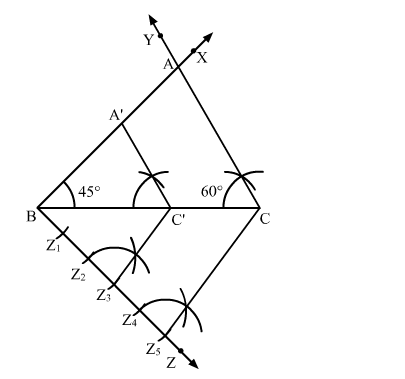# Construct a ∆ABC in which BC = 8 cm, ∠B = 45º and ∠C = 60º.`
Question:

Construct a $\triangle \mathrm{ABC}$ in which $\mathrm{BC}=8 \mathrm{~cm}, \angle \mathrm{B}=45^{\circ}$ and $\angle \mathrm{C}=60^{\circ} .$ Construct another triangle similar to $\triangle \mathrm{ABC}$ such that its sides are $\frac{3}{5}$ of the corresponding sides of

∆ABC.

Solution:

Steps of Construction

Step 1. Draw a line segment BC = 8 cm.

Step 2. At B, draw ">XBC = 45º.

Step 3. At C, draw ">YCB = 60º. Suppose BX and CY intersect at A.

Thus, ∆ABC is the required triangle.

Step 4. Below BC, draw an acute angle ">ZBC.

Step 5. Along BZ, mark five points Z1, Z2, Z3, Zand Zsuch that BZ1 = Z1Z2 = Z2Z3 = Z3Z4 = Z4Z5.

Step 6. Join CZ5.

Step 7. From Z3, draw Z3C' || CZ5 meeting BC at C'.

Step 8. From C', draw A'C' || AC meeting AB in A'.Here, $\triangle \mathrm{A}^{\prime} \mathrm{BC}^{\prime}$ is the required triangle whose sides are $\frac{3}{5}$ of the corresponding sides of $\triangle \mathrm{ABC}$.